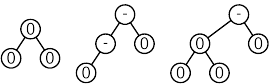5.1 Balancing Rule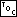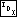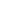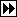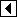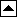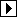A binary search tree is an AVL tree if the difference in height between the subtrees of each of its nodes is between -1 and +1. Said another way, a BST is an AVL tree if it is an empty tree or if its subtrees are AVL trees and the difference in height between its left and right subtree is between -1 and +1.

Here are some AVL trees:

These binary search trees are not AVL trees: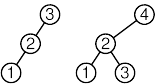In an AVL tree, the height of a node's right subtree minus the height of its left subtree is called the node's balance factor (see balance factor). Balance factors are always -1, 0, or +1. They are often represented as one of the single characters -, 0, or +. Because of their importance in AVL trees, balance factors will often be shown in this chapter in AVL tree diagrams along with or instead of data items. Here are the AVL trees from above, but with balance factors shown in place of data values: